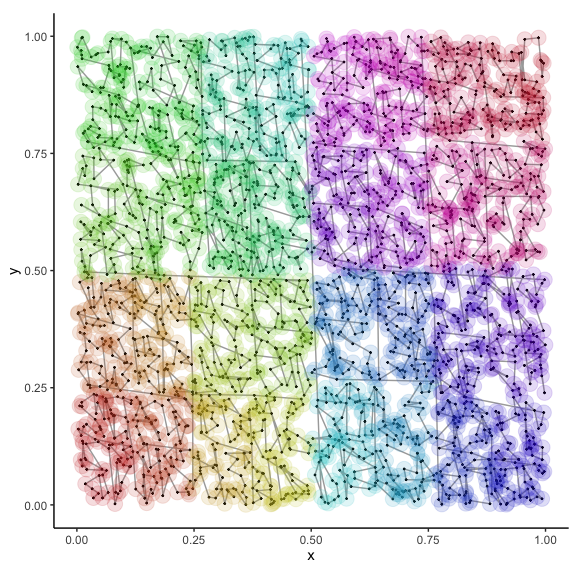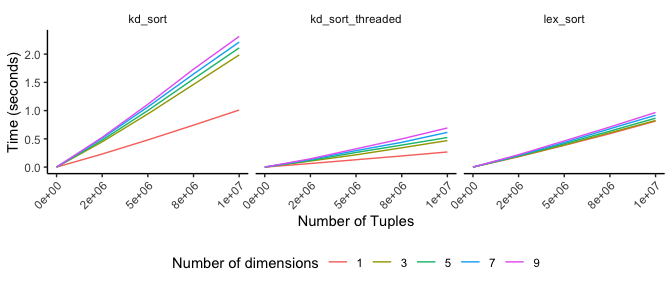# Compact multidimensional sorting and searching with kdtools

## Introduction

Sorting and searching are fundamental operations in computer and data science. The objective of the `kdtools` is to expose a C++ header file implementing efficient sorting and searching methods for multidimensional tuple-like data. The interface mirrors that of the C++ Standard Library sorting and searching functions. It is a single header-only library with no external dependencies other than `std`.

A common approach to improving search performance is to place data into an ordered tree structure, the most common being a binary tree and its variants. Computer textbooks and introductory courses are littered with examples of tree structures, however it has been pointed out by practitioners that many tree-type data structures have considerable storage overhead and poor memory locality. This results from using linked-list-like pointers to connect nodes. In many cases, a fully or partially sorted sequence combined with binary search will outperform pointer-based trees. Indeed, the Standard Library contains a set of algorithms for sorting or partitioning a data sequence and searching within partitioned sequences.

A limitation of the Standard Library algorithms and data structures is that they can only be ordered in a single dimension. Sort keys can possess higher cardinality however comparison operators and ordering are unidimensional. This is suboptimal for data that are intrinsically multidimensional, such as geographical coordinates or other multivariate data. In multiple dimensions, it is preferred to order a sequence of keys such the average distance between neighboring pairs in the sequence is small. There exist a vast array of techniques to accomplish sequence localization; however I will only discuss one known as the kd-tree.

A kd-tree is a type of binary tree that cycles among the dimensions of its stored objects at each level of the tree hierarchy. In the case of spatial data, the root node will contain the median key in the x-direction and each of its daughter nodes will contain the median in the y-direction of their respective partitions. Subsequent daughter nodes will again contain medians in the x-direction, and so on until there are no additional keys to the left or right. Searching a kd-tree involves recursive comparisons, cycling among the dimensions, until a leaf-node is encountered. Most searching operations can be accomplished in logarithmic time complexity yielding an efficient method.

Precisely as an ordinary binary tree can be replaced by a sorted range, a sequence of multidimensional elements can be sorted, in-place if desired, via recursive partitioning following the kd-tree scheme. Divide-and-conquer recursive partitioning is a well-known sorting algorithm and is the basis of the quicksort algorithm. Partitioning a sequence places all elements less than (or more generally meeting some predicate) to the left of a selected key, while all elements greater-than-or-equal-to follow to the right. The kd-sort algorithm presented here simply modifies quicksort to cycle among the dimensions of the stored elements. Searching follows exactly as the kd-tree except that nodes correspond to pivot-elements in the linear array.

Implementation of `kd_sort` enables a set of additional algorithms for searching and nearest neighbor queries. The following table gives the exposed functions and corresponding Standard Library routines.

Function templates SL analog Outcome
kd_sort sort sorts range
kd_is_sorted is_sorted returns `true` if sorted
kd_lower_bound lower_bound finds first element not less than key for all dimensions
kd_upper_bound upper_bound finds first element greater than key for all dimensions
kd_binary_search binary_search returns true if key exists in range
kd_equal_range equal_range returns an iterator pair spanning all occurrences of a key
kd_range_query lower_bound, upper_bound finds all elements not less than lower and less than upper
kd_nearest_neighbor finds nearest neighbor of key
kd_nearest_neighbors, kd_nn_indices finds k-nearest neighbors of key
lex_sort ordinary SL sort using kd_less for comparison

## Sorting

The kd-sort algorithm in the kdtools package is implemented as a C++ function template parameterized by the starting dimension (usually 0) and the iterator type, which will be auto-deduced by the compiler.

``````template <size_t I, typename Iter>
void kd_sort(Iter first, Iter last)
{
using TupleType = iter_value_t<Iter>;
constexpr auto J = next_dim<I, TupleType>;
if (distance(first, last) > 1)
{
auto pred = kd_less<I>();
auto pivot = median_part(first, last, pred);
kd_sort<J>(next(pivot), last);
kd_sort<J>(first, pivot);
}
}``````

The `median_part` function is a thin wrapper around the `nth_element` function from the standard library, which is used to find the median value in the current dimension. Elements to the left of the nth element are not greater than the nth element and elements to the right are not less than the nth element. This allows searching left or right depending on whether the sought value is not greater than the median or not less than the median (ties must search both directions).

Once the first dimension is partitioned, the ranges right and left of the pivot are partitioned, and so on until first and last span a single or no value. The dimension index `I` is incremented at compile-time using template metaprogramming. The `value` member of the templated struct `next_dim` holds the incremented value of `I` that has been wrapped to cycle through successive dimensions of `TupleType`. The result of `kd_sort` is a sequence ordered as if it were inserted into a kd-tree data structure. The following figure illustrates the resulting order in 2-dimensions.Here the sequence order is shown with a continuous color gradient. The resulting patchiness indicates a hierarchical tree-like partitioning of the space providing the desired locality. Placing nearby points in proximity is what allows for efficient searches once the data are sorted. Sort times for vectors of tuple-like objects are relatively efficient, taking roughly twice the time of an ordinary lexicographical sort.In the above results, `lex_sort` is the Standard Library built-in `std::sort` algorithm using `kd_less` as the predicate. The results indicate that `kd_sort` is reasonably fast, requiring roughly twice the time as the Standard Library built-in sorting algorithm. Because of the divide-and-conquer nature of `kd_sort`, it is trivially parallelizable. The threaded version is equal to or faster than the regular sort. Performance comparable to the build-in Standard Library `sort` function indicates that this reference implementation is reasonable.

### Multidimensional comparisons

To ensure the correct behavior in the presence of multiple ties, or even when all keys in one dimension are equal, the `kd_less` predicate implements circular lexicographic comparison. If elements in one dimension are tied, the next dimension is interrogated to break the tie. The comparisons cycle from trailing dimensions to leading dimensions using modulo arithmetic. It is implemented as a recursive, templated function object with the dimension index incremented at compile-time. The recursion is terminated using constexpr if.

``````template <size_t I, size_t K = 0>
struct kd_less
{
template <typename T>
bool operator()(const T& lhs, const T& rhs) const
{
if constexpr (is_last<K, T>) {
return less_nth<I>()(lhs, rhs);
} else {
constexpr auto J = next_dim<I, T>;
return equal_nth<I>()(lhs, rhs) ?
kd_less<J, K + 1>()(lhs, rhs) :
less_nth<I>()(lhs, rhs);
}
}
};``````

The predicates `equal_nth` and `less_nth` compare the \(I^{th}\) element of the tuple. They have specializations for handling pointers to tuple-like object, so all functions can operate on a range over pointers to tuples, allowing arbitrary indexing into the original data.

A more general function object template `kd_compare` that takes an arbitrary binary predicate is also provided by `kdtools`. Some examples applying `kd_less`, including degenerate, all-ties cases, are shown here.

``````j = cbind(sample(1:9))
k = cbind(sample(1:9))
t(kd_sort(j))``````
 1 2 3 4 5 6 7 8 9
``t(kd_sort(cbind(j, k)))``
 2 3 4 1 5 8 9 6 7 4 3 6 8 2 1 5 7 9
``t(kd_sort(cbind(0, j, k)))``
 0 0 0 0 0 0 0 0 0 2 1 3 4 5 6 7 8 9 4 8 3 6 2 7 9 1 5
``t(kd_sort(cbind(j, k, 0)))``
 2 3 4 1 5 8 9 6 7 4 3 6 8 2 1 5 7 9 0 0 0 0 0 0 0 0 0
``t(kd_sort(cbind(0, j, 0)))``
 0 0 0 0 0 0 0 0 0 1 2 3 4 5 6 7 8 9 0 0 0 0 0 0 0 0 0

Notice that `kd_sort` is a variant of the quicksort algorithm in one dimension. It also is not effected by all-ties. The order of subsequent dimensions can be changed when the leading dimension contains all ties, however the sequence is still sorted and can be used for searching.

### Searching

Once data are ordered appropriately, searching can be achieved efficiently. The `kdtools` package provides analogs of the Standard Library `lower_bound`, `upper_bound`, `equal_range` and `binary_search` functions for querying sorted ranges. An additional function, `kd_range_query`, does efficient searching within a boxed region.

The `kd_lower_bound`, `kd_upper_bound` and `kd_equal_range` algorithms behave differently than their unidimensional analogs. This is because the only reasonable definition of multidimensional less is to require less in all dimensions simultaneously. As illustrated below, this leads to cases where some, but not all, elements are less to be included in the interval spanned by `kd_lower_bound`, `kd_upper_bound` and `kd_equal_range`. In other words, in a single dimension, these routines are both necessary and sufficient, whereas in multiple-dimensions, they are necessary, but not sufficient to uniquely identify elements in a boxed region.

In other schemes, such as Morton codes, elaborate subroutines are required to prune the extra elements falling outside the region of interest (see lower-left panel below). This is a result of using a fundamentally unidimensional search on multidimensional data. Instead `kd_sort` implements direct binary search for points falling within a boxed region.

``````template <size_t I,
typename Iter,
typename TupleType,
typename OutIter>
void kd_range_query(Iter first, Iter last,
const TupleType& lower,
const TupleType& upper,
OutIter outp)
{
if (distance(first, last) > 32) {
auto pred = less_nth<I>();
auto pivot = find_pivot<I>(first, last);
constexpr auto J = next_dim<I, TupleType>;
if (within(*pivot, lower, upper)) *outp++ = *pivot;
if (!pred(*pivot, lower)) // search left
kd_range_query<J>(first, pivot, lower, upper, outp);
if (pred(*pivot, upper)) // search right
kd_range_query<J>(next(pivot), last, lower, upper, outp);
} else {
copy_if(first, last, outp, [&](const TupleType& x){
return within(x, lower, upper);
});
}
return;
}``````

Here, each pivot element is tested to see whether it falls within the boxed region, in which case, it is assigned to the output iterator. The template `find_pivot` searches for the partition point whose position may or may not be the middle of the sequence. Leaf tuples are also tested and copied to the output iterator. We check in each dimension whether there might be additional points in the region of interest to the left or right in the array. These are searched recursively until reaching the span falls below a threshold and is more profitably searched via sequential scan. In the following plot, the blue points indicate those not meeting the predicate and the aquamarine points are those where the predicate returns true.